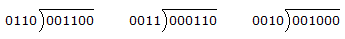# Digital Electronics - Digital Arithmetic Operations and Circuits

### Exercise :: Digital Arithmetic Operations and Circuits - General Questions

41.

Which of the following is correct for full adders?

 A. Full adders have the capability of directly adding decimal numbers. B. Full adders are used to make half adders. C. Full adders are limited to two inputs since there are only two binary digits. D. In a parallel full adder, the first stage may be a half adder.

Answer: Option D

Explanation:

No answer description available for this question. Let us discuss.

42.

Convert each of the signed decimal numbers to an 8-bit signed binary number (two's-complement).
+7        –3        –12

 A. 0000  0111    1111  1101    1111  0100 B. 1000  0111    0111  1101    0111  0100 C. 0000  0111    0000  0011    0000  1100 D. 0000  0111    1000  0011    1000  1100

Answer: Option A

Explanation:

No answer description available for this question. Let us discuss.

43.

What is one disadvantage of the ripple-carry adder?

 A. The interconnections are more complex. B. More stages are required to a full adder. C. It is slow due to propagation time. D. All of the above.

Answer: Option C

Explanation:

No answer description available for this question. Let us discuss.

44.

Solve this binary problem: 01000110 ÷ 00001010 =

 A. 0111 B. 10011 C. 1001 D. 0011

Answer: Option A

Explanation:

No answer description available for this question. Let us discuss.

45.

Divide the following binary numbers.A. 0000  0010    0000  0010    1000  1111 B. 0000  0010    0001  0010    0000  0100 C. 0000  0011    0000  0010    0000  0100 D. 0000  0010    0000  0010    0000  0100

Answer: Option D

Explanation:

No answer description available for this question. Let us discuss.

#### Current Affairs 2021

Interview Questions and Answers